# RD Sharma Class 10 Solutions Chapter 7 Triangles Ex 7.4

## RD Sharma Class 10 Solutions Chapter 7 Triangles Ex 7.4

These Solutions are part of RD Sharma Class 10 Solutions. Here we have given RD Sharma Class 10 Solutions Chapter 7 Triangles Ex 7.4

Other Exercises

Question 1.
(i) In figure, if AB || CD, find the value of x.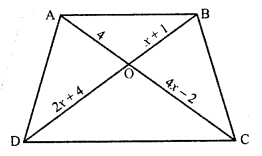(ii) In figure, if AB || CD, find the value of x.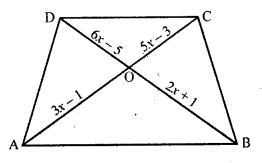(iii) In figure, AB || CD. If OA = 3x – 19, OB = x – 4, OC = x – 3 and OD = 4, find x. (C.B.S.E. 2000C)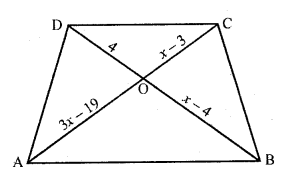Solution:
(i) In the figure, AB || CD
The diagonals of a trapezium divides each other proportionally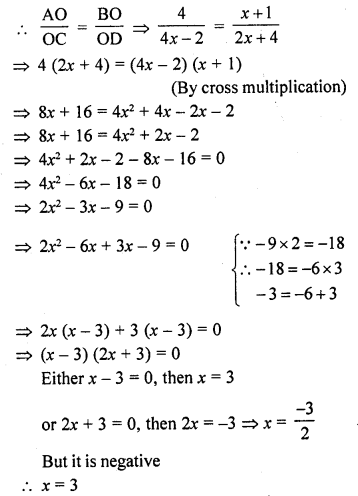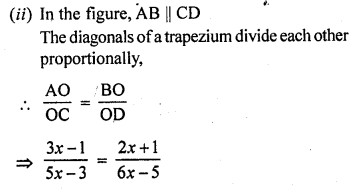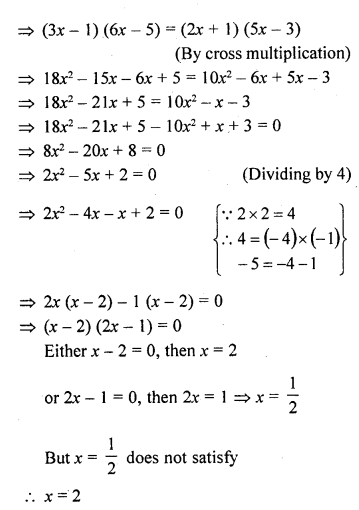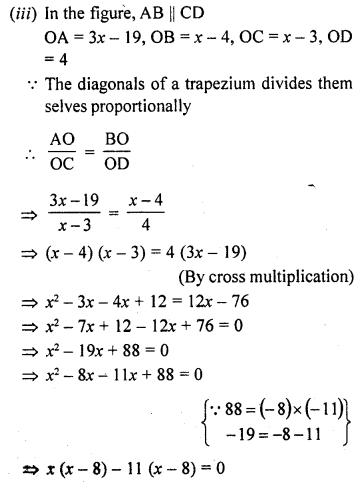=> (x – 8)(x – 11) = 0
Either x – 8 = 0, then x = 8 or x – 11 =0, then x = 11
x = 8 or 11

Hope given RD Sharma Class 10 Solutions Chapter 7 Triangles Ex 7.4 are helpful to complete your math homework.

If you have any doubts, please comment below. Learn Insta try to provide online math tutoring for you.